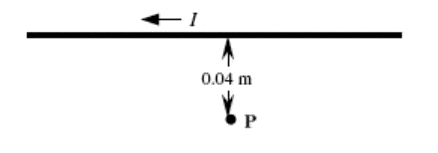# RHR for current problem

outxbreak
1. A long, straight wire is carrying a current of 5.0 A in the direction shown in the
figure. The point P is 0.040 m from the wire.What is the direction of the magnetic field at point P due to the current in the wire?

Answer: out of the plane of the page

I can only see that the current is to the left. I don't understand how to figure out the direction of the magnetic force. :(